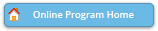#### Abstract Details

 Activity Number: 173 - Novel Approaches to Pedogogy Type: Contributed Date/Time: Monday, July 31, 2017 : 10:30 AM to 12:20 PM Sponsor: Section on Statistical Education Abstract #324983 View Presentation Title: Teaching Logistic Regression Using Ordinary Least Squares in Excel Author(s): Milo Schield* Companies: Augsburg College Keywords: Statistical education ; statistical literacy ; confounding ; GAISE 2016 ; multi-variable methods Abstract: The results of a logistic regression are reported in news stories and journal articles. Logistic regression is a useful tool in modeling data with a binary outcome. If we want students appreciate the value of statistics, we should show them the many tools statistics provides. Yet, logistic regression is seldom - if ever - a part of the intro statistics course. In teaching business statistics, where 70% of classes are taught using Excel, the lack of an Excel Logistic Regression command may seem like a sufficient reason. This paper first reviews how Excel Solver can do multivariate logistic regression using MLE but notes that the process is complicated, time-consuming and non-informative. Although modeling binary outcomes using OLS is not justified theoretically, this paper argues that in many cases the difference is not material. Binary outcomes can be modeled efficiently and effectively using ordinary least squares regression in Excel in three ways. (1) The simplest is to use linear OLS to fit binary outcomes. This approach is quick and simple, but it is limited to those cases where the predicted probabilities of zero and one occur well outside the range of interest. (2) Use OLS to fit a logistic function to grouped data. Grouped data can avoid zeroes and ones that would create infinities in the Log[Odds(pGroup)]. This grouped-data approach introduces students to the logistic function and the need to be careful in interpreting the regression coefficient. Unfortunately, obtaining suitable grouped probabilities may be impossible in multivariate analysis with small samples. (3) This paper introduces the idea of using OLS on the log(odds) of 'nudged data'. Nudging involves replacing the binary values of zero and one with epsilon and one minus epsilon respectively. This new approach gives generally good results for bivariate and multivariate regression. The differences are not generally material in showing students how confounding can influence an association. MLE and Logit-OLS-Nudge are compared in handling a Simpson's reversal with two continuous predictors. This logistic-OLS-nudge approach allows statistical educators to show students how controlling for a confounder can influence - even reverse - an association. Showing this upholds the goal of the 2016 update to the GAISE guidelines: "to give students experience with multivariable thinking" so that they "learn to consider potential confounding factors."

Authors who are presenting talks have a * after their name.